• Publish Your Research/Review Articles in our High Quality Journal for just USD \$99*+Taxes( *T&C Apply)

Offer Ends On### Interest Rate, Exchange Rate and Private Domestic Investment in Nigeria: An Impact Analysis

Ishaku Rimamtanung Nyiputen*, Osang Paul Abijia and Onoyom, Margraret Onwanyi

Corresponding Author: Ishaku Rimamtanung Nyiputen, Department of Economics, Federal University Wukari, Taraba Nigeria.

Received: March 25, 2023 ;    Revised: May 08, 2023 ;    Accepted: May 11, 2023 ;   Available Online: August 10, 2023

Citation:

Views & Citations

### Global Views

• Abstract
• Full Text
• Images
• Tables
• References
• PDF
• Supplementary Files

Everyone concerned about the development of Nigeria is alarmed by the uneven and declining domestic investment. In order to encourage economic growth, it is also crucial to understand and adequately assess a variety of other issues that influence domestic investment. This study looked at the effects of interest rates and exchange rates on domestic investments in Nigeria from 1981 to 2020. Data for the study were sourced from World Development Indicator, and hypotheses were developed to attempt to meet the objectives of version 2020. The ordinary least square's auto-regressive distribution lag was used to assess the objectives of this study. The theoretical framework is based on the monetary transmission mechanism. The variables were stationary at level I and first difference I according to the augmented dickey-Fuller (ADF) unit root test. Even when inflation is not taken into consideration, the variables still exhibit a long-term relationship, according to the ARDL f-bound test. According to the results, Nigeria's low exchange rate, would rising labor force, and reduced or low interest rates will encourage domestic investment. But neither infrastructure nor inflation significantly affect Nigerian domestic investment. The government should thus upgrade the infrastructure it offers, and the monetary authorities should set a fair interest rate and allow for naira depreciation.

Keywords: Interest rate, Exchange rate, Domestic investment

In Nigeria, establishing sustainable development and growth has proven to be rather challenging throughout time. A few of the problems include low levels of savings and investment, uncertainty in monetary and fiscal policy, a decline in the price of crude oil on the international market, excessive interest rates, and inadequate infrastructure development. Nigeria's low investment levels are as a result of the nation's low per capita income, low saving rate, and small capital base. Economic progress is not realized because the marginal or average tendency to consume is so high and the marginal or average tendency to save is so low. For Nigeria's economy to thrive, it is required to increase domestic savings, expand the market, address payment imbalances, reduce foreign loans, use reasonable interest and exchange rates, control inflationary pressure, and take other measures.

People haven't been investing because of the changing currency rates. Investment has fallen, people's standards of living have declined, and the cost of production has increased as a result of this unpredictability and the naira's continual devaluation on the foreign exchange market, which also leads to cost-push inflation. It frequently reduces the ability of non-oil exports to compete internationally and makes forecasting and planning difficult at both the micro and macro levels of the economy.

For instance, corporate level domestic investment in Nigeria underperformed as a result of rising interest rates and volatile exchange rates. To give an example, private domestic investment decreased from 34% of GDP in 1981 to 10% of GDP in 1988. Private domestic investment decreased even further between 1995 and 1996, reaching 7% of GDP. From 1997 through 2003, it varied between 8% and 10% of GDP until reaching an all-time low of 5% of GDP in 2005. Political upheaval, poor infrastructure, and volatile exchange rates may all contribute to this reduction in private domestic investment. Following that, private domestic investment increased to 17% of GDP in 2010 and has since varied between 16% and 15% of GDP.

There are still ways to deal with the ambiguity and variations in investment, though. With the assistance of the IMF, the Nigerian government put the structural adjustment program into action in 1986. The objective was to restructure the economy's patterns of production and consumption by removing price distortions, decreasing dependence on crude oil export, and importing less raw materials and consumer goods. Prior to the start of the structural adjustment program, the monetary authority and central banks fixed interest rates with a specific range between deposit and loan rates. Banks were required to allocate a specific percentage of their financing to the most significant economic sectors. Private domestic investment has not increased consistently despite these efforts. This makes it possible to research the impact of interest and exchange rates on domestic private investment in Nigeria.

LITERATURE REVIEW AND THEORETICAL FRAMEWORK

The marginal efficiency hypothesis of investment was developed by Keynes. The marginal efficiency of investment is the projected rate of return from a certain investment in a capital asset after paying off all of its costs, excluding the rate of investment. Similar to how capital's marginal efficiency affects returns, a capital asset's supply price affects those returns. An asset investment will be made based on the interest rate associated with borrowing money from the market. When interest rates are high, investment levels are low. Low borrowing rates lead to an increase in investment. The MEI therefore links investment to interest rates. Numerous issues are connected to the marginal efficiency investment idea. First, future costs are not considered. The MEI is calculated assuming that all project cash flows may be reinvested to produce an exact rate of return equal to the MEI. But problems always result from this. Another issue is that when there are two fiercely competing systems, MEI is prone to breaking the present standard. Additionally, MEI does not distinguish between borrowing and lending.

Investment Theory based on Neoclassicism

Wale Jorgenson first proposed this theory in 1963. The concept makes the ideal amount of capital stock for a firm clear. It is based on the notion that the rate of investment is determined by how rapidly enterprises increase or decrease their capital stocks to the ideal level. According to this theory, investment and the rise in the economy's capital stock are determined by the marginal product of capital. Its theory of economic development approach is constrained and insufficient. The concept ignores other factors that promote investment.

Investing in Financial Theory

The financial theory of investing was developed by James Duesenberry. It is also known as the cost of capital hypothesis of investment. The accelerator hypotheses contend that a corporation's decision to invest a certain amount is independent on the cost of capital. They falsely believe that the cost of capital for the company, which is independent of the amount of investments it makes, is represented by the market rate of interest. It suggests that companies have free access to financing at market interest rates. When more money is required for investment spending, the price (interest rate) rises. To pay for investment expenses, the corporation may borrow money from the market at whatever interest rates the funds are offered at. One of its shortcomings is that the theory ignores the role of fiscal policy, which is more effective than monetary policy when it comes to encouraging investment. The macroeconomic goal of monetary policy is the one that has the lowest success rates, according to empirical evidence in Kuh and Meyer's theory of investment.

The Mechanism of Monetary Transmission

The traditional monetary transmission mechanism affects interest rates, borrowing costs, levels of physical investment, and aggregate demand through interest rate channels. The term "monetary transmission mechanism" refers to the link between monetary policy and total demand. A conventional interest rate channel is one in which monetary policy affects real investment rates, which in turn affects aggregate demand, consumer spending, and investment spending on new dwellings. The common wisdom holds that easing monetary policy results in falling real interest rates, which cut borrowing costs and encourage significant investment spending, which in turn increases aggregate demand.

The technique is based on two key principles. The first is the ability of the central bank to provide a nominal short-term interest rate. This is undeniably the case, as empirical evidenced by Mojon , who discovered that by regulating short-term nominal interest rates, the central bank can influence both short- and long-term real interest rates. In attempts to understand the transmission from nominal to real short-term interest, price stickiness is a key concept. The relationship between real short-term interest rates and long-term rates is established by the expectation theory. Real long-term interest rates are the average of anticipated future short-term interest rates, according to expectation theory. Changes in the real interest rate will have an effect on investment and consumption decisions, according to the second tenet of the monetary approach.

Review of Empirical Literature

In monetary policy, the interest rate and the exchange rate are important factors. Many researches have examined how monetary policies impact exchange rates. For instance, Mundell claims that one of the primary factors causing uncertainty and swings in small economies is monetary policy [1,2]. The development of elasticity was the subject of the investigation. The trade flow and reserve models, according to the author, were to blame for the fluctuations in the exchange rate in Nigeria over the course of the study.

Green and Villanueva  estimated the effect of macroeconomic conditions and policies, such as interest rates, on private investment in their examination of a group of rising countries. Their findings show that whereas real GDP growth rates and rates of per capita income growth are positively connected with private investment, interest rates and domestic inflation have a negative impact on the private investment ratio. This is contradictory because only low interest rates have a positive effect on GDP. Interest rates shouldn't, therefore, have a negative impact on investments.

Real exchange rate volatility and private investment have a statistically significant inverse relationship, according to Jayalama's  cross-country examination of the macroeconomic environment and private investment in six Pacific Island countries. Thomas  looked at statistics on trade, real exchange rates, and property rights in his research of 86 developing countries. He arrived to the conclusion that, notwithstanding the importance of criteria like credit availability and the standard of the nation's physical and human infrastructure, exchange rate volatility has a detrimental effect on private investment in sub-Saharan Africa.

Bakare  investigated how Nigerian domestic investment performance was impacted by exchange rate adjustments. Using conventional least square multiple regression analytical methods, the findings show a strong but negative correlation between Nigeria's domestic investment and the nation's floating foreign exchange rate. The study's conclusions and findings support the need for the government to switch from the floating exchange system to purchasing power parity, which is believed by researchers to be more appropriate for determining a realistic exchange rate for the naira and enhancing Nigeria's macroeconomic performance. Furthermore, Ezirim, Edith, Mouoghalu  investigated the relationship between the interest rate and the exchange rate in Nigeria. using the use of a distributed autoregressive lag method. According to the analysis, joint strategies on both variables are best for reaching inflation or exchange rate goals.

In Namibia, Wilson and Sheefemi  examined the connection between interest rates and exchange rates. They employed a multi-variant co-integration with quarterly data from 1993 to 2012. There was no link of co-integration.

Additionally, Ologunde, Elumilade and Saolu T  used linear and log-linear specifications to examine the interest rate strategy and performance of the Nigerian manufacturing sector. They found that, for the study period [1980-2004], linear specifications seemed to produce better regression results. The results showed a negative association between interest rates and exchange rates and a positive correlation between foreign capital and investment in the manufacturing sector.

Jonathan O, Emily G, Kenneth U  investigated the impact of exchange rate variations on private domestic investment in Nigeria using error correction model (ECM) methodologies. The major findings of this study show that declining interest rates and the value of the local currency in Nigeria do not encourage domestic private investment. On the other hand, government spending, infrastructure, and inflation all have a positive effect on domestic investment in Nigeria. The report consequently urges the government to continue setting up a sufficient physical, technological, and financial infrastructure as well as that the monetary authorities adopt suitable policies to increase the value of the naira.

Additionally, David Mautin, Koye Gerry, and Olatunji Shobande  looked into the connection between the interest rate and the exchange rate in Nigeria. To conduct the analysis, vector auto-regression was used [VAR]. The conclusion that there is a long-term relationship between the interest rate and exchange rate suggests that the monetary authorities should keep cooperating to better manage the monetary policy rate in order to avoid repeating exchange rate crises in the economy.

METHODOLOGY

This section goes into the methods utilized to carry out the study. Theoretical underpinnings, model design, data analysis approach, estimation evaluation, testing of hypotheses, type and sources of data, methodologies and procedures, and definition of the model have all been discussed.

The theoretical approach employed in this paper is based on the monetary transmission mechanism. Interest rates, which have an effect on borrowing costs, levels of physical investment, and total demand, are the traditional channels of monetary transmission. The monetary transmission mechanism can be used to describe the relationship between monetary policy and overall demand. An example of a conventional interest rate channel is one in which monetary policy affects real investment rates, which in turn affect investments, spending on new dwellings, consumer spending, and aggregate demand. A loosening of monetary policy results in falling real interest rates, which cut borrowing costs and promote significant investment spending, which in turn raises aggregate demand.

The theory suggests that changes in the real interest rate could have an impact on both consumer and investment expenditure. With increasing interest rate elasticity for both sides, monetary policy stimulus has a greater effect. This is especially plausible for long-term investments like fixed investments, residential property investments, and consumer durable purchasing. Additionally, it is anticipated that if domestic interest rates increase, investors will find locally held deposits to be more enticing than deposits maintained in foreign currencies. Domestic currencies gain value relative to those of other countries as a result of the increase in demand. Therefore, domestic products are more expensive abroad while net exports are decreasing. Since net exports are included in total spending, total demand declines.

Model Specification

As was stated in earlier research, the aim of this study is to determine the long-term links among domestic investments, exchange rates, and interest rates as well as to analyze other factors that affect domestic investments in Nigeria. In order to estimate the study's important parameters, a model that will be used is specified in this section. The model therefore examines the relationships among domestic investment, interest rates, exchange rates, inflation rates, real gross domestic product, infrastructure, and money supply.

The model is described as follows in its functional form:

Where; PDI: Domestic Investment proxy by gross fixed capital formation; INR: Interest Rate; EXCH: Exchange Rate; INF: Inflation Rate; INFRA: Infrastructure proxy by aggregate electricity power consumption; LAB: Labor proxy by population growth rate

Mathematically, the model is specified thus;

PDI = B0 + B1INR + B2EXCH+ B3INF + B4INFRAS + B5PGR

The econometric model is specified as;

PDIt = B0+B1INRt+B2EXCHt +B3INFt+ B4 INFRASt + B5PGR+ Ut

However, in order to prevent a spurious result, the variables were standardized. That is, we obtain their natural logarithm. Thus, it is stated as:

lnPDIt = B0+B1lnINRt+B2lnEXCHt +B3lnINFt+B4 lnINFRASt + B5lnPGR+ Ut

Where PDI: Private Domestic Investment; INR: Interest rate; EXCH: Exchange rate; INF: Inflation rate; INFRAS: Proxy for electric power aggregate electricity consumption [KWh per capita]; LABOUR: The population growth rate is used to proxy this variable; Bo: Intercept term; B1- B5: Coefficient of the regressors; Ut: Error term

Estimation Techniques and Procedures

The Ordinary Least Squares estimate method was used in this investigation (OLS). To check whether data are stationary, one might apply the Augmented Dickey Fuller (ADF) unit root test. In order to test for whether there is long run relationship between the variables under consideration, Auto Regressive Distribution Lag (ARDL) model was used. Serial correlation, heteroscedasticity, multi-collinearity, and model specification post-data analytical tests were performed.

Unit Root Test

Researchers face a number of difficulties when using certain series data in econometric analysis. One of these issues is the stationary nature of time series data, as a non-stationary time series is inevitably going to produce erroneous regression. If a series' mean and variance remain constant throughout time, it is considered to be stationary, and the amount of covariance that exists between two time periods relies only on how far off they are from one another. Unit root testing should be employed to determine stationarity because the majority of time series are non-stationary and hence yield false findings.

In order to make sure that the series of modeled variables has a consistent mean and variance, the Augmented Dickey Fuller (ADF) test is important. The goal of this verification is to protect against erroneous regression that could happen when non-stationary variables are applied to OLS at their level of formation. Additionally, the ADF test corrects for high-order serial correlation by addressing a flaw in the Dickey Fuller test (tau statistics), which was that it did not take the potential of autocorrelation in the term into account.

Model using Auto Regressive Distribution Lag [ARDL]

This study uses Pesaran and Shin's  auto regressive distribution lag technique, which Pesaran  argue is the most suitable since it handles mixtures of 1 and 1 variables more effectively. To put this into practice, we first choose the best lag structure for the ARDL model. Prior to bond testing, we ensure that the model is dynamically stable and that the errors are serially independent. According to the Akaike Information Criterion (AIC), both a one- and three-period lag length model are suitable. The autocorrelation test demonstrates the serial independence of the error terms.

Adjusted R2 and the coefficient of determination (R2)

The explanatory power of the regressors on the regress and is assessed using the R squared (R2) of the coefficient of determination or the measure of goodness of fit. The R2 is the proportion of fluctuations in the regressors that are accounted for by changes in the dependent variable. As a result, the model can explain changes in the regress and to a greater extent the higher the R2 value. This means that if R2 is equal to 1, it signifies that the regressors completely account for the variation in the regress and that the regression line fits the data perfectly. If R2 is zero, then the explanatory variable was unable to account for any of the changes in the dependent variable. The better the model matches the data, the higher and closer the R2 is to one. Note that the adjusted “R2” follows the same logic as above.

Econometric Criteria (Second-Order-Test)

Because it assesses the validity of the statistical criterion's representation of statistical theory, this criterion is also known as a second-order test. In accordance with this standard, we examine the OLS estimates for a variety of econometric situations involving heteroskedasticity and multicollinearity. The OLS presupposes that the explanatory variables are not fully correlated (no multicollinearity), that the error term's variance is constant (Heteroskedasticity), and that the error term is normal. In this work, we tested for serial correlation using the Breusch-Godfrey serial correlation LM test. Heteroskedasticity tests can be performed using the Brush-Godfrey-Ragan statistic, the correlation matrix, the Jaque-Bera statistic, the multicollinearity test, the heteroskedasticity test, and the Ramsey-Reset test. A research hypothesis is tested.

The long run and short run t-test method as well as the ARDL f-bound test will be used to test the proposed research hypothesis T.

Reject Ho: If tcal>ttab, we accept the alternative hypothesis at a 5% level of significance and reject the null hypothesis. Otherwise, do not reject.

The ARDL F-bound test and its critical value at the 5% level of significance will be the basis for the f-test decision. If the f-bound test exceeds the upper bound at the 5% level of significance, Ho will be rejected.

Second-order test for the econometric criterion

Because it assesses the validity of the statistical criterion's representation of statistical theory, this criterion is also known as a second-order test. According to this standard, we test the OLS estimates for various autocorrelation, heteroskedasticity, and multicollinearity problems in econometrics. The OLS presupposes that the explanatory variables are not fully correlated (no multicollinearity), that the error term's variance is constant (Heteroskedasticity), and that the error term is normal. In this study, we tested serial correlation using the Breusch-Godfrey serial correlation LM test, heteroskedasticity using the Brush-Godfrey-Ragan statistic, multicollinearity using the correlation matrix, normality using the Jaher-Bera statistic, and model specification error using the Ramsey-Reset test.

RESULT AND DISCUSSION OF FINDING

This criterion is also known as a second-order test since it evaluates the reliability of the statistical criterion's representation of statistical theory. We analyze the OLS estimates for various economic scenarios incorporating heteroskedasticity and multicollinearity in compliance with this criterion. The OLS assumes the error term is normal, the explanatory variables are not fully correlated (no multicollinearity), and the variance of the error term is constant. The Breusch-Godfrey serial correlation LM test was used in this study to check for serial correlation. The Brush-Godfrey-Ragan statistic, the correlation matrix, the Jaque-Bera statistic, the multicollinearity test, the heteroskedasticity test, and the Ramsey-Reset test can all be used to test for heteroskedasticity. A research theory is examined.

The proposed research hypothesis will be examined using the long run and short run t-test method, as well as the ARDL f-bound test T.

Abandon Ho: If tcal>ttab, we reject the null hypothesis at a level of significance of 5% and accept the alternative hypothesis. If not, don't reject.

The f-test decision will be based on the ARDL F-bound test and its critical value at the 5% level of significance. Ho will be disregarded if the f-bound test goes over the upper bound at the 5% threshold of significance.

Second-order econometric criteria test

This criterion is also known as a second-order test since it evaluates the reliability of the statistical criterion's representation of statistical theory. We test the OLS estimates for various autocorrelation, heteroskedasticity, and multicollinearity problems in econometrics in accordance with this standard. The OLS assumes the error term is normal, the explanatory variables are not fully correlated (no multicollinearity), and the variance of the error term is constant. In this study, heteroskedasticity, multicollinearity, normality, and model specification error were all tested. Serial correlation was tested using the Breusch-Godfrey serial correlation LM test, heteroskedasticity was tested using the Brush-Godfrey-Ragan statistic, multicollinearity was tested using the correlation matrix, and normality was tested using the Jaher-Bera statistic (Table 1).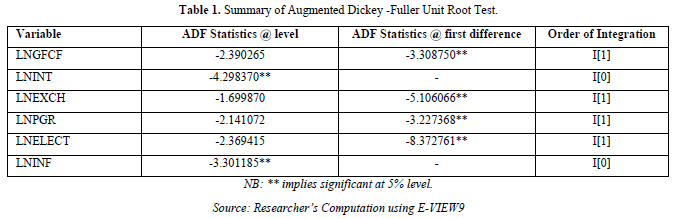The outcome in Table 1 demonstrates that variables including domestic investment (LnGFCF), exchange rate (LnEXCH), labor (LnPGR), and infrastructure (LnELECT) are non-stationary at their level state but become stationary after initial differencing within the context of the Augmented Dickey Fuller Test. This indicates that order one integration of the aforementioned variables, I .

However, the results show that order zero variables, such as the interest rate (LnINT) and inflation rate (LnINF), are stationary at level state .

Integration Test

We move on to test for the presence of a long-run link among the variables based on the findings of the numerous unit root tests that were undertaken. Since there is a combination of I and I variables, the Auto Regressive Distributive (ARDL) Bound testing approach suggested by Pesaran and Shin  and Pesaran  is appropriate.

Given the null hypothesis:

H001=-----------βn= 0

Decision rule:

Case 1: Reject H0 if the F-value is greater than the upper bound

Case 2: Accept H0 if the F-value is less than lower bound

Case 3: Inconclusive if the F-value falls between the lower and upper bounds (Table 2)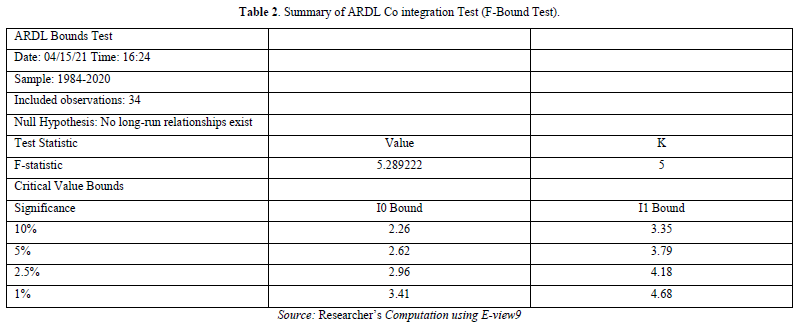The result shows that there is a long-term association between the variables because the F-statistics is bigger than the upper bound at a 5% level of significance. We then estimate an error correction model to take into consideration the short run dynamics of the models given the long run relationship between the variables in each model. We first estimate the unrestricted ARDL model, where each variable is permitted to enter the model at a given lag length, before estimating the short run version of our model (Table 3).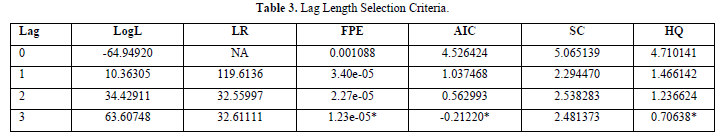The ARDL model is estimated based on the lag length selected using the Akaike Criterion (AIC). The maximum lag length as shown by AIC is three.

Evaluation of Estimates

The existence of long-run relationship between the dependent variable and the independent variables informs the need to estimate both the long-run and short- run versions of our model. The long-run estimates are evaluated based on the following criteria (Tables 4 & 5).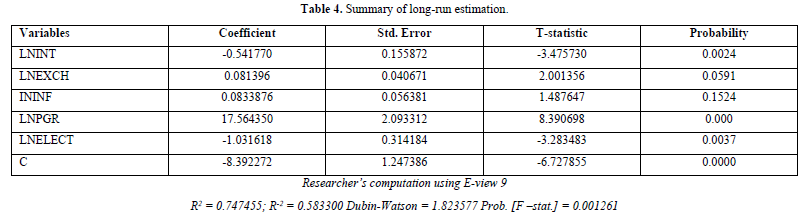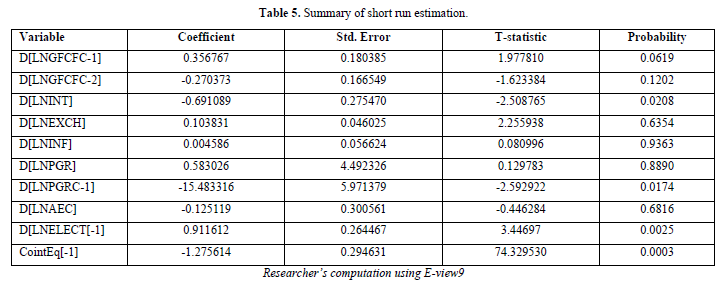Economic Criterion [a Priori Expectation]

Here, we start by determining if the independent variables match their a priori predictions.

At 5% critical values, the interest rate (LnINT) has a statistically significant negative influence on domestic investment (LnGFCF). According to the findings, domestic investment will decrease by roughly 0.542 percent as a result of an average interest rate increase of one percent, maintaining the influence of all other variables constant. This outcome is consistent with the tenets of economic theory because it applies to Nigeria and a fall in interest rates will encourage investment.

At 5 percent critical values, the exchange rate (LnExch) has a statistically significant beneficial influence on domestic investment. The outcome demonstrates that, while controlling for the impact of other variables, a one percent average appreciation in exchange rates will result in a 0.814 percent increase in the value of the naira. This outcome is consistent with economic theory because a depreciation will lower a currency's worth relative to other foreign currencies while an appreciation will increase the value of a local currency.

Furthermore, domestic investment in Nigeria shows a positive and statistically insignificant association with inflation (LnINF) at 5 percent critical values. According to the findings, domestic investment in Nigeria will grow by around 0.083 percent for every 1 percent increase in inflation, holding other factors constant. The outcome does not match the a priori economic prediction. Because investment will always be distorted in the short term by an increase in inflation. Long-term, however, things are quite different since rising nominal wages will prevent inflation from driving away domestic investment. The aforementioned scenario is valid in Nigeria, but because it is so negligible over the long term, it cannot be used to form a forecast.

Additionally, at 5 percent critical values, the influence of labor, as measured by the population growth rate (LnPGR), on domestic investment is positive and statistically significant. According to the findings, domestic investment will increase by around 17.56 percent for every one percent increase in labor. Accordingly, more labor leads to more domestic investment in Nigeria. Economic theory is consistent with this empirical reality. Furthermore, domestic investment will increase by an amount greater than proportionate to a 1% increase. The amount of electricity produced for consumption has not been sufficient.

Furthermore, there is a negative and statistically significant association between domestic investment and infrastructure as measured by total electricity usage (LnELECT). According to the coefficient, domestic investment will decrease by around 1.031 percent for every 1 percent increase in electricity usage. A rise in electricity consumption is supposed to encourage domestic investment, hence this empirical reality contradicts economic theory. Nigeria's electricity use, however, has not been at its best. Nigeria's electricity production hasn't been sufficient to affect domestic investment. Additionally, there are areas in Nigeria without access to electricity. And in certain areas of Nigeria, there is no electricity. It didn't live up to the a priori anticipation because of this (Table 6).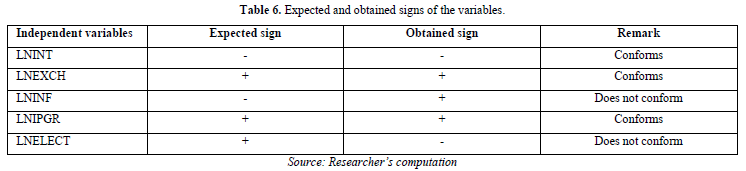First-order test is a statistical criterion

A number of different coefficients of determination. The explanatory factors, according to table (Table 3), explain around 74.5 percent of the variation in this domestic investment, according to the R2 statistic. Under does not account for 25.5%. The corrected R-2, which indicates that the independent value accounts for around 58.3 percent of the variation in domestic investment, further supports the model's goodness of fit.

T-Test

The outcome of the long-run estimate (Table 3) reveals that while inflation was statistically insignificant because its P-value was greater than inflation at the 5 percent level of significance, interest rates, exchange rates, labor costs, and infrastructure costs were statistically significant.

F-Test

According to the general F-value, with a contact value of 5%, all partial coefficients are not simultaneously equal to zero and start to become statistically significant.

Second-order test for econometric criteria

To support the source of the CNLRM assumption that our model is appropriate for, the projected long-run model is further assessed. The model is assessed using various econometric standards, including the Ramsey-Reset Test, the Normality Test, and the LM Serial Correlation Test.

Serial Correlation Test

To check for serial correlation errors in a regression model, the Breusch-Godfrey serial correlation LM test was used (Table 7).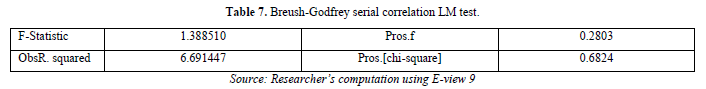The Breusch-Godfrey final correlation LM Test indicates that there is no serial correlation since the probability of the F-statistic for the test is greater than the 5% significance level. This implies the acceptance of the null hypothesis of no serial correlation in the model.

In Test for model specification

The Ramsey-Reset test is used to test whether the model adopted   in this study is correctly specified (Table 8).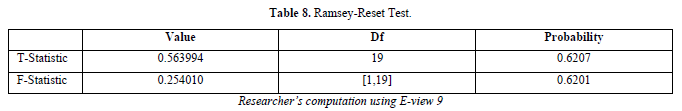We accept the null hypothesis and reject the alternative hypothesis because the probability of the t-statistic and f-statistic having a level of significance greater than 5% indicates that the model is correctly defined. It has no issues with misspecification.

Normality Test

The goal of the normalcy test is to determine whether the population we are using for this investigation is roughly distributed. The normality test is performed using Jaque-Bera statistics (Table 1), and since the P-values are less than 5%, the population is considered to be normally distributed.

Assessment of the Short-Run Model

We present our model's short-run estimates in Table 6. The outcome demonstrates that, at a 5% level of significance, the error correction term is correctly signed with a value of 1.27 and statistically significant. This demonstrates that there are long-term relationships among the model's variables. The outcome shows that the models about 100% disequilibrium is correlated within a year.

According to their probability values, one era of domestic investment (LnGFCF) has a positive and statistically significant impact on present domestic investment, while two periods of domestic investment (LnGFCF) have a negative and statistically negligible impact.

mortgage rate with the exception of electricity consumption, which is not statistically significant, the Ume period lag of PGR and Electricity consumption demonstrated a negative link and statistically significant influence on domestic investment in the short run.

Except for inflation, which is statistically insignificant as indicated by their p-values, the link between the exchange rate, inflation, and one period of power usage was positive.

DISCUSSION OF FINDINGS

In this study, the effects of interest rates and currency exchange rates on private domestic investment in Nigeria between 1981 and 2020 are investigated. The long-term results showed that the interest rate (LnINT), exchange rate (LnEXCH), and population growth rate (LnPGR) all met prior forecasts. However, both infrastructure and inflation (measured by total electricity usage) deviate from their respective a priori predictions. The long-run association between domestic investment and the independent variables (exchange rate, interest rate, population growth rate, and aggregate electricity power consumption) is confirmed by the Auto Regressive Distribution Lag (ARDL) bound test, with the exception of inflation.

Therefore, interest rates in Nigeria have a bad and statistically significant link with domestic investment. According to the coefficient, a 1% increase in interest rates will typically result in a 0.542 decline in domestic investment in Nigeria. In other words, a rise in interest rates causes a drop in domestic investment. Given that a rise in interest rates will result in a decline in domestic investment, the empirical situation is consistent with the postulate of economic theory.

The relationship between the exchange rate and domestic investment is favorable and consistent. According to the coefficient, a one percent average increase in exchange rates will cause a 0.0814 percent increase in domestic investment in Nigeria. This indicates that an increase in the value of the naira will benefit her trading partners. Furthermore, because of the inelastic nature of the naira's coefficient, a 1% increase in the value of the currency results in a less than proportionate increase in domestic investment. The empirical situation is consistent with economic theory. An increase in the value of a local currency in comparison to that of other foreign nations, given a steady exchange rate, will strengthen a local economy.

In Nigeria, domestic investment and inflation are positively correlated, though statistically insignificantly. According to the long-term coefficient, a 1% increase in inflation will result in a 0.083% increase in domestic investment in Nigeria. Since the inflation rate's coefficient is inelastic, an increase in inflation will result in a smaller than proportional increase in domestic investment.

Economic theory is in conflict with this. Because domestic investment will decline when inflation rises in the short term. In Nigeria, this theory is untrue. The first is that as inflation rises, employees start to demand higher wages (a rise in nominal wage). The increase in nominal earnings won't completely counteract inflation, though.

Domestic investment and population growth rate are positively and statistically significantly correlated. According to the coefficient, domestic investment in Nigeria will increase by roughly 17.564 percent for every one percent increase in the population growth rate. Accordingly, a growing population will result in more labor, which will encourage domestic investment. The empirical situation is consistent with economic theory. Furthermore, domestic investment will increase at a faster rate than proportionate to a 1% increase in population.

Lastly, total electricity power consumption in Nigeria, has a significant negative association with domestic investment. According to the coefficient, domestic investment will decrease by around 1.031 for every percentage point increase in total power usage. This empirical reality deviates from economic theory because infrastructure (increased electricity usage) is supposed to encourage domestic investment. The optimal level of electricity usage has not, however, been reached in Nigeria. Nigeria's domestic investment has not increased as a result of its high electricity usage. Furthermore, electricity is not available in several areas of Nigeria. The enormous population of Nigeria also complies with this nonconformity because the electricity produced does not satisfy consumption needs.

SUMMARY OF FINDINGS

According to the Augmented Dickey Fuller (ADF) test, while the currency rate, population growth rate, electricity consumption, and domestic investment are stationary at first difference, the inflation and interest rates are stationary at their current levels. Additionally, the outcome of the co-integration test demonstrates the existence of a long-run equilibrium relationship between each independent variable and the dependent variable (domestic investment) across the research period (1981-2020). The OLS result shows that a trustworthy goodness of fit was attained by the estimated model. According to the results of the t-test, domestic investment in Nigeria is significantly impacted by inflation and exchange rates but not by interest rates, population growth rates, or total electricity consumption. Additionally, the interest rate, exchange rate, and population growth rate all meet their presumptions. Furthermore, there is no serial correlation, according to the Breusch-Godfrey serial correlation LM test. Additionally, the model is appropriately stated, according to the Ramsey-Reset test.

CONCLUSION

In this study, the effects of interest rates and currency exchange rates on domestic investment in Nigeria from 1981 to 2020 were investigated. It used a method known as auto regressive distributive lag. According to the empirical data from this study, interest rates, currency rates, and labor (as measured by the rate of population increase) all have a long-term impact on domestic investment. This suggests that they influence Nigerian domestic investment in the long run. On the other hand, there is no long-term correlation between inflation and domestic investment. Only short-term investment distortion results from inflation. However, in order to maintain public confidence in the Nigerian currency, the monetary authority must guarantee stability. Additionally, infrastructure that was designed to encourage domestic investment has not done so effectively (as measured by total electricity usage). The total electricity power consumption in Nigeria, has a significant negative association with domestic investment. This empirical reality deviates from economic theory because infrastructure (increased electricity usage) and it’s supposed to encourage domestic investment, but in this study, revise is the case, the question is what could be the reasons for the deviations from the economic theory.  This will be the focus for next research work in these areas. Therefore, based on the results of this study, targeted efforts should be made to target the interest rate and the currency rate, while also taking other factors that affect domestic investment in Nigeria into consideration.

RECOMMENDATION

It is required to offer a set of policy recommendations that would be applicable to the Nigerian economy based on the results of this research. As a result, the research provides the following possibilities:

1. To start, domestic investment is negatively correlated with infrastructure. This suggests that domestic investment has not been encouraged by infrastructure. Infrastructure should be better provided by the government in order to encourage investment and create jobs.
2. In order to support domestic investment, the monetary authorities should maintain a stable exchange rate and a low interest rate. Furthermore, given that it helps Nigeria's domestic investment expand, labor should be promoted even more.
3. The monetary authorities should properly control money circulation since inflation has a direct and negligible relationship with domestic investment. In order to prevent repeated exchange rate crises in Nigeria, the monetary authority should further make a concentrated effort to regulate the monetary policy rate more efficiently.
1. Mandell RA (1973a) Uncommon Arguments for Common Currencies: In H. G. Johnson & A. K., Swebodo (eds.), The Economics of Common Currencies. London: Allen and Union.
2. Mundel RA (1973b) A plan for European Currency in H. G. Johnson & A. K. Suroboda (Eds.), The Economics of Common Currencies. London: Allen and Union.
3. Gujarati DN (2003) Basic Econometrics (5thEd.) New York: McGraw-Hill Inc.
4. Thomas RL (1997) Modern Econometrics, An Introduction, Sydney: Addison Wesley, pp: 452.
5. Bakare AS (2011) The Consequences of Foreign Rate Reforms on the Performances of Private Domestic Investment in Nigeria. Int J Econ Manag Sci 1(1): 25-31.
6. Ezirim CB, Edith AH, Mouoghalu MI (2012) Autoregressive Distributed Lag Analysis of interdependence between Inflation and Exchange Rate in Sub-Saharan Africa. The IABAPAD Conf Proceed 9(2): 1082-1093.
7. Ologunde A, Elumilade D, Saolu T (2006) Stock Market Capitalization and Interest Rate in Nigeria-A time series Analysis. Int Res J Finance Econ A: 154-167.

No Files Found